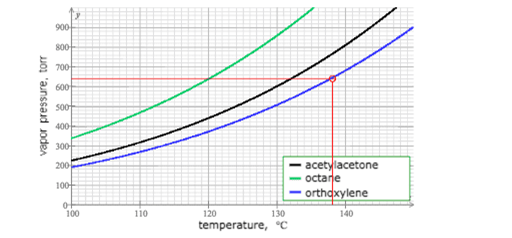# Problem: This graph shows how the vapor pressure of three liquids varies with temperature:Use the graph to answer the following questions:Which liquid is the most volatile? Which is the least volatile? What is the normal boiling point of each liquid? Suppose a beaker of octane is put inside a sealed tank containing octane gas at 112°C and 281. torr. After ten minutes, will there be more liquid in the beaker, less liquid, or the same amount?

###### FREE Expert Solution

The higher the vapor pressure the higher the volatility.

88% (129 ratings)###### Problem Details

This graph shows how the vapor pressure of three liquids varies with temperature:Use the graph to answer the following questions:

Which liquid is the most volatile?

Which is the least volatile?

What is the normal boiling point of each liquid?

Suppose a beaker of octane is put inside a sealed tank containing octane gas at 112°C and 281. torr. After ten minutes, will there be more liquid in the beaker, less liquid, or the same amount?